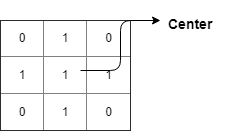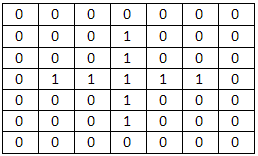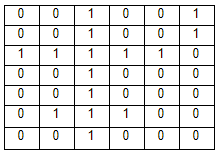# Game in a Binary Gridshahriarsust13SUST Inter University Pro...
Limits 2s, 512 MB

Binary grid indicates to a grid that contains only 0s and 1s in its cells. You can find a plus(+) symbol in a binary grid.

Following grid contains a plus(+) symbol.You can form a plus symbol by choosing some cells from a grid if the chosen cells satisfy all of the following conditions:

• Number of 1s in each four direction must be same.
• Each cell containing 1 (except the center) must have 0 in both side. For the cells in horizontal line both side means adjacent upper and lower cell, for the cells in vertical line both side means adjacent left and right cell.

Weight of a plus symbol means the distance between the center and furthest cell containing 1. Weight of the above plus(+) symbol is 1. Whenever you make a plus(+) symbol, your objective is to maximize its weight. Same cell can be part of multiple plus symbol.

Following grid contains a plus symbol of weight 2.Score of a grid is the sum of weight of all plus symbols in it.

Score of the following grid is 3 (weight of plus symbol centered in (3, 3) cell is 2 and weight of plus symbol centered in (6, 3) cell is 1)Bob has received a binary grid from his friend as a birthday gift. But he has lost the data of this grid. He has become sad, because he didn’t calculate the score of his grid. Only thing he can remember is the length and width of the grid. So he is interested in knowing the expected score of the grid if it is filled up randomly. You have a good friendship with Bob. So you want to help Bob by calculating the expected score of his grid.

You will be given the length and width of a binary grid. Your task is to calculate the expected score of the grid if it is filled up randomly. Assume that 0s and 1s are distributed in the grid with equal probability.

## Input

First line contains an integer $T$ ($1 ≤ T ≤ 10^3$), denotes the number of test cases. Then $T$ line follows, Each line contains two integers $N$ and $M$ ($1 ≤ N$, $M ≤ 10^5$), denotes the length and width of the grid respectively.

## Output

For each case, output the case number and the expected score in a single line.

If the answer is $\frac{P}{Q}$, then print it as $P \times Q^{-1} \space \text{mod} \space 1000000007$.

Here, $P$ is an integer greater than or equal to 0, $Q$ is an integer greater than 0.

## Sample

InputOutput
1
3 3

Case 1: 142578126


### Submit